Click in-app to access the full platform documentation for your version of DataRobot.

# Time series feature derivation¶

The following tables document the feature derivation process—operators used and feature names created—that create the time series modeling dataset. For additional information, see the descriptions of:

## Process overview¶

When deriving new features, DataRobot passes each feature through zero or more preprocessors (some features are not preprocessed), then passes the result though one or more extractors, and then finally through postprocessors.

Preprocessors are only run—although this step can be skipped—for target, date, and text columns (no feature columns):

dataset --> preprocessor --> extractor --> postprocessor --> final

Feature columns move from input to extractor or postprocessor:

dataset --> extractor --> postprocessor --> final

dataset --> extractor --> final

More detailed, DataRobot:

1. Applies automatic feature transformation on date features during EDA1. These features are excluded from the EDA2 feature derivation process described below; only the original undergoes the process (i.e., transformed features are not further transformed).
2. Applies a preprocessor (e.g., CrossSeriesBinaryPreprocessor, NextEventType, Transform, and others).
3. Creates an "intermediate feature" for the target, date, or text features—a feature where preprocessing was applied but will not be complete until application of the post-processing operation. For example, `Sales (log)` is an intermediate step to the final `Sales (log) (diff) (14 day min)` and by itself is not a valid feature.
4. Uses an extractor step to consume the input from either the original dataset or the intermediate feature. The postprocessor (next step) consumes this output as input.
5. Applies postprocessing to the results of the extractor, creating the "final feature" to use for modeling.

See the visual representation of feature generation for a quick reference.

### Feature reference¶

The following provides a general overview of the derivation process.

A sample input dataset:

Date Target
1/1/20 1
2/1/20 2
3/1/20 3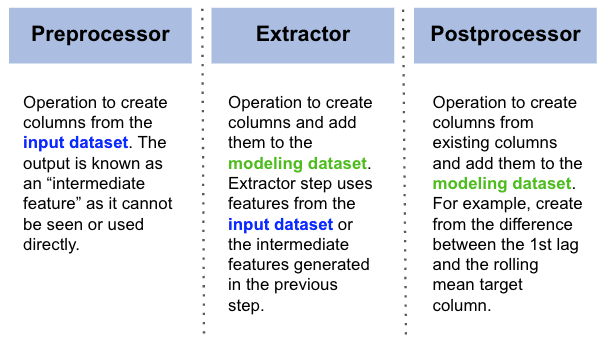The resulting time series modeling dataset:

Date (actual) Target (actual) Forecast distance
1/1/20 1 1
2/1/20 2 1
3/1/20 3 1
1/1/20 1 2
2/1/20 2 2
3/1/20 3 2

Example of target-derived feature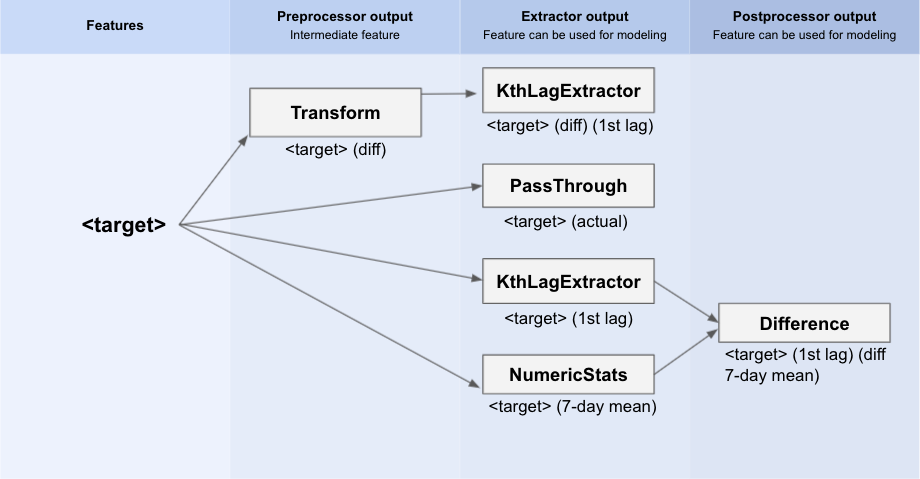Example of numeric feature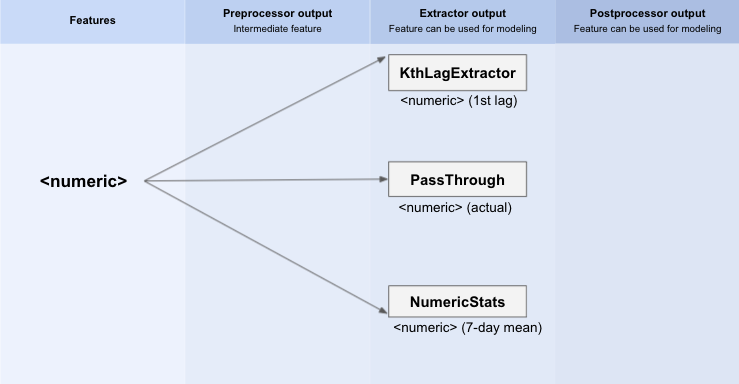Example of categorical feature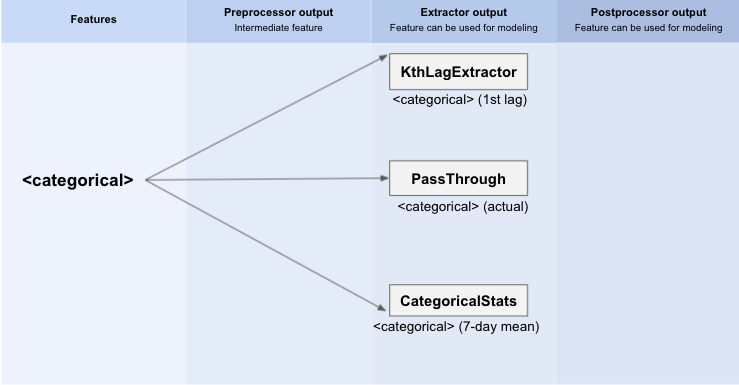Example of text feature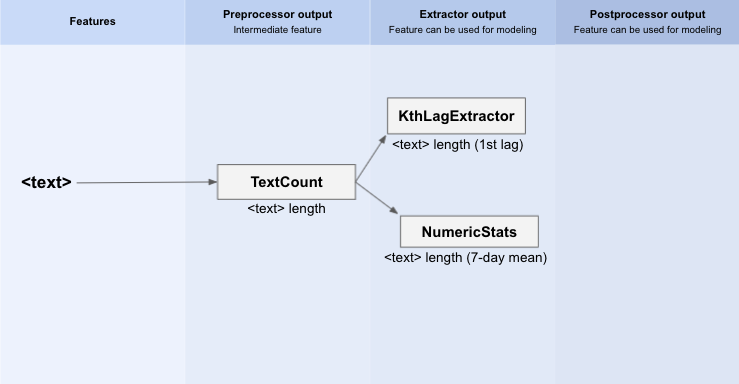Example of date feature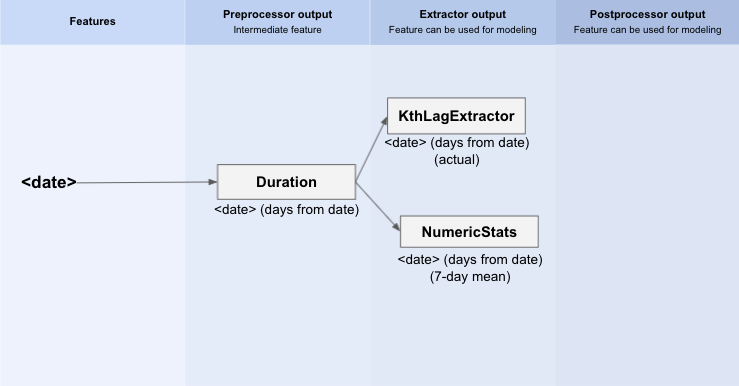## Feature types¶

Feature derivation acts on features based on their type. The examples and explanations below use these variables (for example, `<target>`) to describe the interactions.

Component Description
(intermediate)
(final)
The feature selected at project start as the feature to predict.
(final) Any feature or target column from the dataset that is not of type date or text. Processing is the same as that done to the target if the feature is numeric; if the feature is categorical, there are differences (noted in the tables below). DataRobot does not apply preprocessing to non-target features.
(intermediate)
(final)
The primary date/time feature selected to enable time-aware modeling at project start.
(intermediate)
(final)
Any date feature, other than automatically transformed features during EDA1, that is not a primary date/time feature.
(intermediate) A text column.

The tables include information on:

• Feature name patterns—the feature type followed by the pattern tag ("actual" if the feature is from the original uploaded dataset). This is the resulting feature name after all transformations are complete (for example: `<target> (diff)`).
• Tags—characteristics of the feature.
• Examples of the post-processed feature.

## Intermediate features¶

The sections below detail the intermediate features created for target, primary date, date, and text features.

The sections below list each name pattern for target features.

`<target> (log)`

Description: A log-transformed target.

Project type: Regression, multiplicative trend

Tags:

• Target-derived
• Numeric
• Multiplicative

Example(s):

``````    sales (log) (naive latest value)
sales (log) (diff) (1st lag)
sales (log) (7 day diff) (35 day max)
sales (log) (1 month diff) (2nd lag)
``````

`<target> (diff)`

Description: A diff-transformed target, created by calculating the difference between the current value and the previous single time step value. Time step is based on the interval in the uploaded dataset. Example: A quarterly dataset has a time step of 3 months.

Project type: Regression, non-stationary

Tags:

• Target-derived
• Numeric
• Stationarity

Example(s):

``````sales (diff) (1st lag)
sales (diff) (7 day mean)
``````

`<target> (<period> diff)`

Description: A diff-transformed target, created by calculating the difference between the current value and the previous value.

Project type: Regression, seasonality

Tags:

• Target-derived
• Numeric
• Seasonal

Example(s):

``````sales (7 day diff) (1st lag)
sales (7 day diff) (14 day mean)
``````

`<target> (1 month diff)`

Description: A diff-transformed target, created by calculating the difference between the current value and the previous month (same day of month) value.

Project type: Regression, intramonth seasonality

Tags:

• Target-derived
• Numeric
• Seasonal

Example(s):

``````sales (1 month diff) (35 day mean)
sales (1 month diff) (1st lag)
``````

`<target> (1 month match end diff)`

Description: A diff-transformed target, created by calculating the difference between the current value and the previous month (aligned to the end of the month) value.

Project type: Regression, intramonth seasonality

Tags:

• Target-derived
• Numeric
• Seasonal

Example(s):

``````sales (1 month match end diff) (2nd lag)
sales (1 month match end diff) (35 day max)
``````

`<target> (1 month match weekly diff)`

Description: A diff-transformed target, created by calculating the difference between the current value and the previous month (aligned to the week of the month and weekday) value.

Project type: Regression, intramonth seasonality

Tags:

• Target-derived
• Numeric
• Seasonal

Example(s):

``````sales (1 month match weekly diff) (3th lag)
sales (1 month match weekly diff) (35 day mean)
``````

`<target> (1 month match weekly diff from end)`

Description: A diff-transformed target, created by calculating the difference between the current value and the previous month (aligned to the weekday and the "week of the month from the end of the month") value.

Project type: Regression, intramonth seasonality

Tags:

• Target-derived
• Numeric
• Seasonal

Example(s):

``````sales (1 month match weekly diff from end) (2nd lag)
sales (1 month match weekly diff from end) (35 day min)
``````

`<target> (total)`

Description: Total target, for the given time, across all series.

Project type: Cross series regression, total aggregation

Tags:

• Target-derived
• Numeric
• Cross series

Example(s):

``````sales (total) (2nd lag)
sales (total) (35 day mean)
sales (total) (3rd lag) (diff 35 day mean)
sales (total) (7 day diff) (35 day mean)
``````

`<target> (weighted total)`

Description: Weighted total target, for the given time, across all series.

Project type: Cross series regression, total aggregation, user-specified weights

Tags:

• Target-derived
• Numeric
• Cross series
• Weighted

Example(s):

``````sales (weighted total) (2nd lag)
sales (weighted total) (35 day mean)
sales (weighted total) (3rd lag) (diff 35 day mean)
sales (weighted total) (7 day diff) (35 day mean)
``````

`<target> (<groupby> total)`

Description: Total target, for the given time, across all series within the same user-specified group.

Project type: Cross series regression, total aggregation, user-specified groupby feature

Tags:

• Target-derived
• Numeric
• Cross series

Example(s):

``````sales (region total) (2nd lag)
sales (region total) (35 day mean)
sales (region total) (3rd lag) (diff 35 day mean)
sales (region total) (7 day diff) (35 day mean)
``````

`<target> (<groupby> weighted total)`

Description: Weighted total target, for the given time, across all series within the same user-specified group.

Project type: Cross series regression, total aggregation, user-specified groupby feature, user-specified weights

Tags:

• Target-derived
• Numeric
• Cross series
• Weighted

Example(s):

``````sales (region weighted total) (2nd lag)
sales (region weighted total) (35 day mean)
sales (region weighted total) (3rd lag) (diff 35 day mean)
sales (region weighted total) (7 day diff) (35 day mean)
``````

`<target> (average)`

Description: Target average, for the given time, across all series.

Project type: Cross series regression, average aggregation

Tags:

• Target-derived
• Numeric
• Cross series

Example(s):

``````sales (average) (2nd lag)
sales (average) (35 day mean)
sales (average) (3rd lag) (diff 35 day mean)
sales (average) (7 day diff) (35 day mean)
``````

`<target> (weighted average)`

Description: Weighted target average, for the given time, across all series.

Project type: Cross series regression, average aggregation, user-specified weights

Tags:

• Target-derived
• Numeric
• Cross series
• Weighted

Example(s):

``````sales (weighted average) (2nd lag)
sales (weighted average) (35 day mean)
sales (weighted average) (3rd lag) (diff 35 day mean)
sales (weighted average) (7 day diff) (35 day mean)
``````

`<target> (<groupby> average)`

Description: Target average, for the given time, across all series within the same group.

Project type: Cross series regression, average aggregation, user-specified cross-series groupby feature

Tags:

• Target-derived
• Numeric
• Cross series

Example(s):

``````sales (region average) (2nd lag)
sales (region average) (35 day mean)
sales (region average) (3rd lag) (diff 35 day mean)
sales (region average) (7 day diff) (35 day mean)
``````

`<target> (<groupby> weighted average)`

Description: Weighted target average, for the given time, across all series within the same group.

Project type: Cross series regression, total aggregation, user-specified groupby feature and weights

Tags:

• Target-derived
• Numeric
• Cross series
• Weighted

Example(s):

``````sales (region weighted average) (2nd lag)
sales (region weighted average) (35 day mean)
sales (region weighted average) (3rd lag) (diff 35 day mean)
sales (region weighted average) (7 day diff) (35 day mean)
``````

`<target> (proportion)`

Description: Numeric target that specifies the proportion of the target across all series.

Project type: Cross series regression, total aggregation, nonnegative target, sufficiently consistent series presence across timestamps

Tags:

• Target-derived
• Numeric
• Cross series

Example(s):

``````sales (proportion) (1st lag)
sales (proportion) (14 day mean)
sales (proportion) (30 day max) (diff 7 day mean)
sales (proportion) (7 day diff) (1st lag)
sales (proportion) (7 day diff) (30 day min)
``````

`<target> (weighted proportion)`

Description: Numeric target that specifies the weighted proportion of the target across all series.

Project type: Cross series regression, total aggregation, nonnegative target, sufficiently consistent series presence across timestamps, user-specified weights

Tags:

• Target-derived
• Numeric
• Cross series
• Weighted

Example(s):

``````sales (weighted proportion) (1st lag)
sales (weighted proportion) (14 day mean)
sales (weighted proportion) (30 day max) (diff 7 day mean)
sales (weighted proportion) (7 day diff) (1st lag)
sales (weighted proportion) (7 day diff) (30 day min)
``````

`<target> (<groupby> proportion)`

Description: Numeric target that specifies the proportion of the target across all series within the same group.

Project type: Cross series regression, total aggregation, nonnegative target, sufficiently consistent series presence across timestamps, user-specified cross-series groupby feature

Tags:

• Target-derived
• Numeric
• Cross series

Example(s):

``````sales (region proportion) (naive latest value)
sales (region proportion) (2nd lag)
sales (region proportion) (7 day mean)
sales (region proportion) (1st lag) (diff 7 day mean)
sales (region proportion) (7 day diff) (1st lag)
sales (region proportion) (7 day diff) (30 day min)
``````

`<target> (<groupby> weighted proportion)`

Description: Numeric target that specifies the weighted proportion of the target across all series within the same group.

Project type: Cross series regression, total aggregation, nonnegative target, sufficiently consistent series presence across timestamps, user-specified cross-series groupby feature and weights

Tags:

• Target-derived
• Numeric
• Cross series
• Weighted

Example(s):

``````sales (region weighted proportion) (naive latest value)
sales (region weighted proportion) (2nd lag)
sales (region weighted proportion) (7 day mean)
sales (region weighted proportion) (1st lag) (diff 7 day mean)
sales (region weighted proportion) (7 day diff) (1st lag)
sales (region weighted proportion) (7 day diff) (30 day min)
``````

`<target> (total equal <label>)`

Description: Total target that equals

Project type: Cross-series classification, total aggregation

Tags:

• Target-derived
• Binary
• Cross series

Example(s):

```````is_zero_sales (total equal 1) (1st lag)`
``````

`<target> (weighted total equal <label>)`

Description: Weighted total target-equals-`<label>` boolean flag, for a given time, across all series.

Project type: Cross-series classification, total aggregation, user-specified weights

Tags:

• Target-derived
• Binary
• Cross series
• Weighted

Example(s):

``````is_zero_sales (weighted total equal 1) (1st lag)
is_zero_sales (weighted total equal 1) (1st lag) (diff 35 day mean)
``````

`<target> (<groupby> total equal <label>)`

Description: Total target-equals-`<label>` boolean flag, for a given time, across all series and within the same group.

Project type: Cross-series classification, total aggregation, user-specified groupby feature

Tags:

• Target-derived
• Binary
• Cross series

Example(s):

``````is_zero_sales (region total equal 1) (1st lag)
is_zero_sales (region total equal 1) (1st lag) (diff 35 day mean)
``````

`<target> (<groupby> weighted total equal <label>)`

Description: Weighted total target-equals-`<label>` boolean flag, for a given time, across all series within the same group.

Project type: Cross-series classification, total aggregation, user-specified cross-series groupby feature and weights

Tags:

• Target-derived
• Binary
• Cross series
• Weighted

Example(s):

``````is_zero_sales (region weighted total equal 1) (1st lag)
is_zero_sales (region weighted total equal 1) (1st lag) (diff 35 day mean)
``````

`<target> (fraction equal <label>)`

Description: Average target-equals-`<label>` (also called fraction) boolean flag, for a given time, across all series.

Project type: Cross-series classification, average aggregation

Tags:

• Target-derived
• Binar
• Cross series

Example(s):

``````is_zero_sales (fraction equal 1) (1st lag)
is_zero_sales (fraction equal 1) (1st lag) (diff 35 day mean)
``````

`<target> (weighted fraction equal <label>)`

Description: Weighted average target-equals-`<label>` (also called fraction) boolean flag, for a given time, across all series.

Project type: Cross-series classification, average aggregation, user-specified weights

Tags:

• Target-derived
• Binary
• Cross series
• Weighted

Example(s)

``````is_zero_sales (weighted fraction equal 1) (3rd lag)
is_zero_sales (weighted fraction equal 1) (3rd lag) (diff 35 day mean)
``````

`<target> (<groupby> fraction equal <label>)`

Description: Average target-equals-`<label>` (also called fraction) boolean flag, for a given time, across all series within the same group.

Project type: Cross-series classification, average aggregation, user-specified cross-series groupby feature

Tags:

• Target-derived
• Binary
• Cross series

Example(s):

``````is_zero_sales (region fraction equal 1) (3rd lag)
is_zero_sales (region fraction equal 1) (3rd lag) (diff 35 day mean)
``````

`<target> (<groupby> weighted fraction equal <label>)`

Description: Weighted average target-equals-`<label>`(also called fraction) boolean flag, for a given time, across all series within the same group.

Project type: Cross-series binary, average aggregation, user-specified cross-series groupby feature and weights

Tags:

• Target-derived
• Binary
• Cross series
• Weighted

Example(s):

``````is_zero_sales (region weighted fraction equal 1) (3rd lag)
is_zero_sales (region weighted fraction equal 1) (3rd lag) (diff 35 day mean)
``````

`<target> (is zero)`

Description: Boolean flag that indicates whether the target equals zero (used by zero-inflated tree-based models).

Project type: Regression, minimum target equals zero

Tags:

• Target-derived
• Numeric
• Zero-inflated

Example(s):

``````sales (is zero) (1st lag)
sales (is zero) (7 day fraction equal 1)
sales (is zero) (naive binary) (35 day fraction equal 1)
sales (is zero) (1st lag) (diff 35 day mean)
``````

`<target> (nonzero)`

Description: Replaces zero target value with missing value (used by zero-inflated tree-based models).

Project type: Regression, minimum target equals zero

Tags:

• Target-derived
• Numeric
• Zero-inflated

Example(s):

``````sales (nonzero) (log) (1st lag) (diff 35 day mean)
sales (nonzero) (7 day max) (log) (diff 35 day mean)
sales (nonzero) (35 day average baseline) (log)
``````

`<target> (<time_unit> aggregation)`

Description: Aggregates target data to a higher time unit (used by temporal hierarchical models).

Project type: Regression

Tags:

• Target-derived
• Numeric

Example(s):

`sales (week aggregation) (actual)`

`<target> (weighted <time_unit> aggregation)`

Description: Weighted target data, aggregated to a higher time unit (used by temporal hierarchical models).

Project type: Regression, user-specified weights

Tags:

• Target-derived
• Numeric

Example(s):

`sales (weighted week aggregation) (actual)`

The sections below list each name pattern for the primary date/time feature.

`<primary_date> (previous calendar event type)`

Description: Value of the previous calendar event. For example, if the calendar file has two events—Christmas and New Year—all observations between December 25 and January 1 will have previous calendar event type equal to “Christmas.” All observations between January 1 and December 25 will have feature equal to “New Year.” If there is no previous value, the feature will be null.

Tags:

• Date
• Calendar

Example(s):

`date (previous calendar event type) (actual)`

`<primary_date> (next calendar event type)`

Description: Value of the next calendar event. For example, if the calendar file has two events—Christmas and New Year—all observations between December 25 and January 1 will have the next calendar event type equal to “New Year.” All observations between January 1 and December 25 will have feature equal to “Christmas”.

Tags:

• Date
• Calendar

Example(s):

`date (previous calendar event type) (actual)`

`<primary_date> (calendar event type <N> day(s) before)`

Description: Feature that specifies a calendar event N days before the date of the observation. For example, if the observation date is December 27, the feature `date (calendar event type 2 days before) (actual)` will be equal to "Christmas." Feature `date (calendar event type 1 days before) (actual)` will be null.

If event types are not provided in the calendar file, this feature will take (1) or (0) values, specifying whether there is a calendar event N days before.

Tags:

• Date
• Calendar

Example(s):

``````date (calendar event type 1 day before) (actual)
date (calendar event type 2 days before) (actual)
``````

`<primary_date> (calendar event type <N> day(s) after)`

Description: Feature that specifies a calendar event N days after the date of the observation. For example, if the observation date is December 23, feature `date (calendar event type 2 days after) (actual)` will be equal to "Christmas." Feature `date (calendar event type 3 days after) (actual)` will be null.

If event types are not provided in the calendar file, this feature will take (1) or (0) values specifying whether there is a calendar event N days after.

Tags:

• Date
• Calendar

Example(s):

`date (days from previous calendar event) (actual)`

`<primary_date> (<time_unit>(s) from previous calendar event)`

Description: Numeric feature that specifies the number of time units since a previously known calendar event. Time units depend on the dataset time step (e.g., for daily datasets, time units are in days). For example, if the observation date is December 28, this feature will be equal to 3 (in days).

Tags:

• Date
• Calendar

Example(s):

``````date (calendar event type 1 day after) (actual)
date (calendar event type 2 days after) (actual)
``````

`<primary_date> (<time_unit>(s) to next calendar event)`

Description: Numeric feature that specifies the number of time units until the next known calendar event. Time units depend on the dataset time step (e.g., for daily datasets, time units are in days). For example, if the observation date is December 30, this feature will be equal to 5 (in days).

Tags:

• Date
• Calendar

Example(s):

``````date (calendar event type 1 day after) (actual)
date (calendar event type 2 days after) (actual)
``````

`<primary_date> (calendar event type)`

Description: Specifies calendar events happening on the same date as the observation. For example, for observation an on December 25, the feature will be equal to “Christmas.” For December 26, the feature will be null.

Tags:

• Date
• Calendar

Example(s):

`date (calendar event type) (actual)`

`<primary_date> (calendar event)`

Description: Specifies whether there is a calendar event on the date. Values are (1) if there is a calendar event on the same date as observation, otherwise (0).

Tags:

• Date
• Calendar

Example(s):

`date (calendar event) (actual)`

`<primary_date> (hour of week)`

Description: Equals (day of week * 24 + hour) of the primary date. Result enumerates hours from beginning of the week to the end of the same week.

Project type: Detected weekly seasonality, 24-hour seasonality

Tags:

• Date

Example(s):

`date (hour of Week) (actual)`

`<primary_date> (common event)`

Description: Specifies whether the primary date is expected to be there or not. For example, for a Monday-to-Friday dataset, all the samples with primary date within (inclusive) Monday to Friday are true. Samples with weekend primary date will have the value of false.

Project type: Regular missing of sample on certain day-of-week or hour-of-day (e.g., Monday to Friday dataset)

Tags:

• Date

Example(s):

`date (common event) (actual)`

The sections below list each name pattern for date—non primary date/time—features.

`<date> (<time_unit>s from <primary_date>)`

Description: Numeric feature that specifies the number of time units from the input date feature to the primary date/time. Output of this preprocessor is a numeric feature. Input is a date feature.

Project type: Any, with minimum one non-low-info and non-primary date/time feature (at least one feature that fulfills both conditions).

Tags:

• Date

Example(s):

``````due_date (days from date) (1st lag)
due_date (days from date) (7 day mean)
``````

The section below lists each name pattern for text features.

`<text> Length`

Description: Numeric feature that specifies the number of characters in a text column. Output of this preprocessor is a numeric feature. Input is a text feature.

Project type: Numeric, minimum one non-low-info text input

Tags:

• Text

Example(s):

``````(description Length) (1st lag)
(description Length) (7 day mean)
``````

## Final features¶

The sections below detail the final features created using target-only, feature/target/intermediate, primary date, and date features during the feature engineering process.

The sections below list each name pattern for features that can be either a target, a non-target feature, or an intermediate feature.

`<feature_or_target_or_intermediate> (actual)`

Description: Simple passthrough feature that, for a specific date, has the same value as in the raw dataset. These features are considered to be known in advance and can be copied as-is from the raw to the derived dataset. For non-target features, it is used when the feature is available at prediction time. Examples are date, date-derived, calendar, or user specified known-in-advance (a priori) features. For the target or derived target column, it is used as the target to fit the model.

Tags:

• Calendar
• Date-derived
• Target
• Target-derived

Example(s):

``````sales (actual)
date (actual)
date (Month of Year) (actual)
date (calendar event) (actual)
sales (actual)
sales (week aggregation) (actual)
``````

`<feature_or_target_or_intermediate> (<N> lag)`

Description: Feature extracts the N th most recent value in the feature derivation window. The minimum number of lags for any project is 1. For projects with a zero forecast distance (FDW=[-n, 0] and FW=), the last value in the feature derivation window is the value at the forecast point and so the first lag is equivalent to the actual value known at the forecast point.

Tags:

• Lag

Example(s):

`````````
sales (2nd lag)
sales (region average) (1st lag)
sales (region total) (4th lag)
sales (diff 7 day) (2nd lag)
```
``````

`<feature_or_target_or_intermediate> (<window> <time_unit> <categorical_method>)`

Description: Feature extracts categorical statistics within the most recent `<window> <time_unit>` of the feature derivation window. The categorical statistics include "most_frequent" (returns item with the highest frequency), "n_unique" (returns number of unique values) and "entropy" (measure of uncertainty).

Tags:

• Category

Example(s):

``````product_type (7 day most_frequent)
product_type (7 day n_unique)
product_type (7 day entropy)
``````

`<feature_or_target_or_intermediate> (same <matching_period>) (<window> <time_unit> <categorical_method>)`

Description: Feature extracts the categorical statistics of the same period within the most recent `<window> <time_unit>` of the feature derivation window. The categorical statistics include "most_frequent" (returns item with the highest frequency), "n_unique" (returns number of unique values) and "entropy" (measure of uncertainty). For example, the feature `product_type (same weekday) (35 day entropy)` computes `product_type` entropy of weekdays equal to forecast point over the last 5 weeks.

Tags:

• Category

Example(s):

``````product_type (same weekday) (35 day most_frequent)
product_type (same weekday) (35 day n_unique)
product_type (same weekday) (35 day entropy)
``````

`<feature_or_target_or_intermediate> (<window> <time_unit> <fraction>)`

Name patterns:

`<feature_or_target_or_intermediate> (<window> <time_unit> fraction empty)`

`<feature_or_target_or_intermediate> (<window> <time_unit> fraction equal <label>)`

Description: Feature computes the fraction of `<feature> equals <label>`. If `<label>` is an empty string, `<feature> equals <label>` becomes `fraction empty` within the most recent `<window> <time_unit>` of the feature derivation window. For example, `is_raining (7 day fraction empty)` computes the fraction of the `is_raining` feature equal to an empty string over the last 7 days.

Tags:

• Binary

Example(s):

``````is_holiday (35 day fraction equal True)
is_raining (7 day fraction equal empty)
``````

`<feature_or_target_or_intermediate> (same <matching_period>) (<window> <time_unit> <fraction>)`

Name patterns:

`<feature_or_target_or_intermediate> (same <matching_period>) (<window> <time_unit> fraction empty)`

`<feature_or_target_or_intermediate> (same <matching_period>) (<window> <time_unit> fraction equal <label>)`

Description: Feature computes the fraction of `<feature> equals <label>`. If `<label>` is an empty string, `<feature> equals <label>` becomes `fraction empty` of the same period within the most recent `<window> <time_unit>` of the feature derivation window. For example, `is_raining (same weekday) (35 day fraction equal True)` computes the fraction of the `is_raining` feature equal to true over the last 35 days.

Tags:

• Binary

Example(s):

``````is_raining (same weekday) (35 day fraction equal True)
is_holiday (same weekday) (35 day fraction equal empty)
``````

`<feature_or_target_or_intermediate> (<window> <time_unit> <method>)`

Description: Feature computes the numerical statistic `<method>` within the most recent `<window> <time_unit>` of the feature derivation window. The numeric statistics include "max," "min," "mean," "median," "std," and "robust zscore."

Tags:

• Numeric

Example(s):

``````sales (7 day max)
sales (7 day min)
sales (7 day mean)
sales (7 day median)
sales (7 day std)
``````

`<feature_or_target_or_intermediate> (same <matching_period>) (<window> <time_unit> <method>)`

Description: Feature computes the numerical statistic `<method>` of the same period within the most recent `<window> <time_unit>` of the feature derivation window. For example, the feature `sales (same weekday) (35 day mean)` computes the mean value of `sales` on the same weekday over the last 35 days.

Tags:

• Numeric

Example(s):

``````sales (same weekday) (35 day max)
sales (same weekday) (35 day min)
sales (same weekday) (35 day mean)
sales (same weekday) (35 day median)
``````

The sections below list each name pattern for target-only features.

`<target> (naive or match) <strategy>`

Name patterns:

`<target> (naive latest value)`

`<target> (naive <period> seasonal value)`

`<target> (naive 1 month seasonal value)`

`<target> (match end of month) (naive 1 month seasonal value)`

`<target> (match weekday from start of month) (naive 1 month seasonal value)`

`<target> (match weekday from end of month) (naive 1 month seasonal value)`

Description: Feature selects value from history to forecast the future based on different strategies. Naive latest prediction uses the latest history value to forecast the rows in the forecast window. Naive seasonal prediction extracts the previous season's target value in the history to forecast. For example, for a given Monday-Friday dataset, naive latest prediction on Monday uses the target value of last Friday as the forecast for Monday. For the naive 7-day prediction, it uses the target value of last Monday. If a multiplicative trend is detected on the dataset, the naive prediction is in log scale.

Tags:

• Numeric
• Naive/baseline

Example(s):

``````sales (naive lastest value)
sales (naive 7 day seasonal value)
sales (naive 1 month seasonal value)
sales (match end of month) (naive 1 month seasonal value)
sales (match weekday from start of month) (naive 1 month seasonal value)
sales (match weekday from end of month) (naive 1 month seasonal value)
sales (log) (naive lastest value)
sales (log) (naive 7 day seasonal value)
sales (log) (naive 1 month seasonale value)
sales (log) (match end of month) (naive 1 month seasonal value)
sales (log) (match weekday from start of month) (naive 1 month seasonal value)
sales (log) (match weekday from end of month) (naive 1 month seasonal value)
``````

`<target> (last month <strategy>)`

Name patterns:

`<target> (last month average baseline)`

`<target> (last month weekly average)`

`<target> (match end of month) (last month weekly average)`

Description: Feature computes the previous month average target value, or previous month weekly average target value, with respect to the forecast point.

For example, `sales (last month average baseline)` computes the average target value in the previous month, `sale (last month weekly average)` computes the weekly average target value of the same week in the previous month, `sales (match end of month) (last month weekly average)` computes the weekly average target value of the same week (aligned to the end of month) in the previous month. If multiplicative is detected in the dataset, log transform is applied after average value is computed.

Tags:

• Numeric
• Naive/baseline

Example(s):

``````sales (last month average baseline)
sales (last month weekly average)
sales (match end of month) (last month weekly average)
sales (last month average baseline) (log)
sales (last month weekly average) (log)
sales (match end of month) (last month weekly average) (log)
``````

`<target> (last month <fraction_strategy>)`

Name patterns:

`<target> (last month fraction empty)`

`<target> (match end of month) (last month weekly fraction empty)`

`<target> (last month fraction equal <label>)`

`<target> (match end of month) (last month weekly fraction equal <label>)`

Description:

Feature computes the fraction of the boolean flag that compares whether target equals

Tags:

• Binary

Example(s):

``````sales (last month fraction empty)
sales (match end of month) (last month weekly fraction empty)
sales (last month fraction equal True)
sales (match end of month) (last month weekly fraction equal True)
``````

`<target> (last month weekly <fraction>)`

Name patterns:

`<target> (last month weekly fraction empty)`

`<target> (last month weekly fraction equal <label>)`

Description: Feature computes the fraction of the boolean flag that compares whether target equals

Tags:

• Binary

Example(s):

``````sales (last month weekly fraction empty)
sales (last month weekly fraction equal True)
``````

`<target> (naive binary) (match_and_fraction )`

Name patterns:

`<target> (naive binary) (last month fraction empty)`

`<target> (naive binary) (last month weekly fraction empty)`

`<target> (naive binary) (match end of month) (last month weekly fraction empty)`

`<target> (naive binary) (last month fraction equal <label>)`

`<target> (naive binary) (last month weekly fraction equal <label>)`

`<target> (naive binary) (match end of month) (last month weekly fraction equal <label>)`

Description: Feature has the same value as the one without "naive binary" (for example, `<target> (naive binary) (last month fraction empty)` has the same value as `<target> (last month fraction empty)`). The distinction is that it can be used for naive binary predictions.

Tags:

• Binary
• Naive/baseline

Example(s):

``````is_raining (naive binary) (last month fraction empty)
is_raining (naive binary) (last month weekly fraction empty)
is_raining (naive binary) (match end of month) (last month weekly fraction empty)
is_raining (naive binary) (last month fraction equal True)
is_raining (naive binary) (last month weekly fraction equal True)<
is_raining (naive binary) (match end of month) (last month weekly fraction equal True)
``````

`<target> (naive binary) (<window> <time_unit> <fraction>`

Name patterns:

`<target> (naive binary) (<window> <time_unit> fraction empty)`

`<target> (naive binary) (<window> <time_unit> fraction equal <label>)`

Description: Feature has the same value as a feature without "naive binary" (for example, `<target> (naive binary) (<window> <time_unit> fraction empty)` has the same value as `<target> (<window> <time_unit> fraction empty)`). The distinction is that it can be used for naive binary predictions.

Tags:

• Binary
• Naive/baseline

Example(s):

``````is_raining (naive binary) (35 day fraction equal True)
is_raining (naive binary) (35 day fraction equal empty)
``````

`<target> (<window> <time_unit> mean baseline)`

Description: Feature is the same as `<target> (<window> <time_unit> mean)`. The distinction is that it can be used for naive predictions.

Tags:

• Numeric

Example(s):

`sales (7 day mean baseline)`

`<target> (last month weekly average baseline)`

Description: Feature computes the average between ```<target> (last month weekly average)``</span> and <code> (match end of the month) (last month weekly average)`</span>.</br> For example,sales (last month weekly average)is the average ofsales (last month weekly average)(which is the average of sales on last month/same week) andsales (match end of the month) (last month weekly average)` (which is the average of sales on last month/same week, week count starts from end of the month).```

Tags:

• Numeric

Example(s):

`sales (last month weekly average baseline)`

`<primary_date> (<naive_boolean>)`

Name patterns:

`<primary_date> (No History Available)`

`<primary_date> (naive <period> prediction is missing)`

`<primary_date> (naive 1 month prediction is missing)`

`<primary_date> (match end of month) (naive 1 month prediction is missing)`

`<primary_date> (match weekday from start of month) (naive 1 month prediction is missing)`

`<primary_date> (match weekday from end of month) (naive 1 month prediction is missing)`

Description: Boolean flag feature that specifies whether its corresponding naive prediction is missing. For example, a 7-day naive prediction on this Friday is missing if the shop was closed last Friday. In this case, the boolean feature value is true on this Friday. Each of these boolean features is related to different naive predictions. `<primary_date> (No History Available)` is related to naive latest predictions whereas the rest of the boolean features are related to different types of naive seasonal predictions.

Tags:

• Numeric
• Multiseries

Example(s):

``````date (No History Available)
date (naive 7 day prediction is missing)
date (naive 1 month prediction is missing)
date (match end of month) (naive 1 month prediction is missing)
date (match weekday from start of month) (naive 1 month prediction is missing)
date (match weekday from end of month) (naive 1 month prediction is missing)
``````

`<target_derived> (diff <strategy>)`

Name patterns:

`<target_derived> (diff <window> <time_unit> mean)`

`<target_derived> (diff last month weekly mean)`

`<target_derived> (diff last month mean)`

Description: Feature computes the difference between the target-derived and baseline features. For example: • `sales (1st lag) (diff 7 day mean)` is the difference between `sales (1st lag)` and `sales (7 day mean baseline)``sales (35 day max) (diff last month weekly mean)` is the difference between `sales (35 day meax)` and `sales (last month weekly average baseline)``sales (7 day mean) (diff last month mean)` is the difference between `sales (7 day mean)` and `sales (last month average baseline)`.

Tags:

• Numeric

Example(s):

``````sales (1st lag) (diff 7 day mean)
sales (35 day max) (diff last month weekly mean)
sales (7 day mean) (diff last month mean)
``````

`<date> (<time_unit>s between 1st forecast distance and last observable row)`

Description: Feature computes the time delta (in terms of integer number of time units) between the date/time of the first forecast distance and the date/time of the last row in the feature derivation window.

Tags:

• Numeric
• Row-based

Example(s):

`date (days between 1st forecast distance and last observable row)`

Updated October 26, 2021